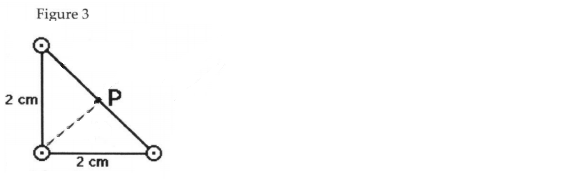# Problem: Three very long, straight, parallel wires each carry currents of 4 A, directed out of the page in the drawing in Figure 3. The wires pass through the vertices of a right isosceles triangle of side 2 cm. What is the magnitude of the magnetic field at point P at the midpoint of the hypotenuse of the triangle?

🤓 Based on our data, we think this question is relevant for Professor Li's class at FIU.

###### Problem Details

Three very long, straight, parallel wires each carry currents of 4 A, directed out of the page in the drawing in Figure 3. The wires pass through the vertices of a right isosceles triangle of side 2 cm. What is the magnitude of the magnetic field at point P at the midpoint of the hypotenuse of the triangle?